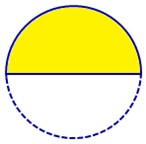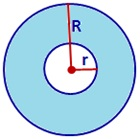©Ms. Garcia Math Name: ID: Quiz: Grade: Circle sectors π (Pi) = 3.14 3.1 3 ? 1)a What is the radius? yd b What is the diameter? yd c What is the perimeter? yd 2)a What is the area? cm² b What is the perimeter? cm (A quarter a circle is normally referred to as a quadrant) 3)a What is the radius? in b What is the perimeter? in 4)a What is the area? m² (An annulus is the area between two concentric circles)